In :
%matplotlib inline


# APSG tutorial - structural geology module for Python¶

APSG defines several new python classes to easily manage, analyze and visualize orientation structural geology data. Base class Vec3 is derived from numpy.array class and offers several new method which will be explained in following examples.

## Basic usage¶

APSG module could be imported either into own name space or into active one for easier interactive work

In :
from apsg import *
settings['figsize'] = (10, 8)


### Basic operations with vectors¶

Instance of vector object Vec3 could be created from any iterable object as list, tuple or array

In :
u = Vec3([1, -2, 3])
v = Vec3([-2, 1, 1])


For common vector operation we can use standard mathematical operators or special methods using dot notation

In :
u + v

Out:
V(-1.000, -1.000, 4.000)
In :
u - v

Out:
V(3.000, -3.000, 2.000)
In :
3*u - 2*v

Out:
V(7.000, -8.000, 7.000)

Its magnitude or length is most commonly defined as its Euclidean norm and could be calculated using abs

In :
abs(v)

Out:
2.449489742783178
In :
abs(u + v)

Out:
4.242640687119285

For dot product we can use multiplication operator * or dot method

In :
u*v

Out:
-1
In :
u.dot(v)

Out:
-1

For cross product we can use operator ** or method cross

In :
u**v

Out:
V(-5.000, -7.000, -3.000)
In :
u.cross(v)

Out:
V(-5.000, -7.000, -3.000)

To project vector u onto vector v we can use method proj

In :
u.proj(v)

Out:
V(0.816, -0.408, -0.408)

To find angle (in degrees) between to vectors we use method angle

In :
u.angle(v)

Out:
96.26395271992722

Method rotate provide possibility to rotate vector around another vector. For example, to rotate vector u around vector v for 45°

In :
u.rotate(v, 45)

Out:
V(2.248, 0.558, 2.939)

## Classes Lin and Fol¶

To work with orientation data in structural geology, APSG provide two classes derived from Vec3 class. There is Fol class to represent planar features by planes and Lin class to represent linear feature by lines. Both classes provide all Vec3 methods, but they differ in way how instance is created and how some operations are calculated, as structural geology data are commonly axial in nature.

To create instance of Lin or Fol class, we have to provide dip direction and dip, both in degrees

In :
Lin(120, 60), Fol(216, 62)

Out:
(L:120/60, S:216/62)

or we can create instance from Vec3 object using aslin and asfol properties

In :
u.aslin, u.asfol

Out:
(L:297/53, S:117/37)

### Vec3 methods for Lin and Fol¶

To find angle between two linear or planar features we can use method angle

In :
l1 = Lin(110, 40)
l2 = Lin(160, 30)
l1.angle(l2)

Out:
41.59741268003547
In :
p1 = Fol(330, 50)
p2 = Fol(250, 40)
p1.angle(p2)

Out:
54.69639932197533

We can use cross product to construct planar feature defined by two linear features

In :
l1**l2

Out:
S:113/40

or to construct linear feature defined as intersection of two planar features

In :
p1**p2

Out:
L:278/36

Cross product of planar and linear features could be used to construct plane defined by linear feature and normal of planar feature

In :
l2**p2

Out:
S:96/53

or to find perpendicular linear feature on given plane

In :
p2**l2

Out:
L:276/37

To rotate structural features we can use method rotate

In :
p2.rotate(l2, 45)

Out:
S:269/78

## Classes Pair and Fault¶

To work with paired orientation data like foliations and lineations or fault data in structural geology, APSG provide two base Pair class and derived Fault class. Both classes are instantiated providing dip direction and dip of planar and linear measurements, which are automatically orthogonalized. If misfit is too high, warning is raised. The Fault class expects one more argument providing sense of movement information, either 1 or -1.

To create instance of Pair class, we have to provide dip direction and dip of planar and linear feature, both in degrees

In :
p = Pair(120, 40, 162, 28)
p

Out:
P:118/39-163/30
In :
p.misfit

Out:
3.5623168411508175

Planar and linear features are accessible using fol and lin properties

In :
p.fol, p.lin

Out:
(S:118/39, L:163/30)

To rotate Pair instance we can use rotate method

In :
p.rotate(Lin(45, 10), 60)

Out:
P:314/83-237/61

Instantiation of Fault class is similar, we just have to provide argument to define sense of movement

In :
f = Fault(120, 60, 110, 58, -1)  # -1 for normal fault
f

Out:
F:120/59-110/59 -

Note the change in sense of movement after Fault rotation

In :
f.rotate(Lin(45, 10), 60)

Out:
F:312/62-340/59 +

To find rotation axis and angle between two Pair instances

In :
pr = p.rotate(Lin(45, 10), 60)
F = p.H(pr)
axis, angle = F.axisangle
print('Rotation axis: {}'.format(axis.aslin))
print('Rotation angle: {:g}°'.format(angle))

Rotation axis: L:45/10
Rotation angle: -60°


For simple fault analyses Fault class also provide p, t, m and d properties to get PT-axes, kinematic plane and dihedra separation plane

In :
f.p, f.t, f.m, f.d

Out:
(L:315/75, L:116/14, S:27/85, S:290/31)

## Group class¶

Group class serve as a homogeneous container for Lin, Fol and Vec3 objects. It allows grouping of features either for visualization or batch analysis

In :
g = Group([Lin(120,60), Lin(116,50), Lin(132,45), Lin(90,60), Lin(84,52)], name='L1')
g

Out:
G:5 Lin (L1)

To simplify interactive group creation, you can use function G

In :
g = G('120 60 116 50 132 45 90 60 84 52', name='L1')
g

Out:
G:5 Lin (L1)

Method len returns number of features in group

In :
len(g)

Out:
5

Most of the Lin, Fol and Vec3 methods could be used for Group as well. For example, to measure angles between all features in group and another feature, we can use method angle

In :
g.angle(Lin(110,50))

Out:
array([11.49989817,  3.85569115, 15.61367789, 15.11039885, 16.3947936 ])

To rotate all features in group around another feature, we can use method rotate

In :
gr = g.rotate(Lin(150, 30), 45)


To show data in list you can use data property

In :
gr.data

Out:
[L:107/35, L:113/26, L:126/30, L:93/26, L:94/18]

Property R gives mean or resultant of all features in group. Note that Lin and Fol are axial in nature, so resultant vector is not reliable. You can use ortensor property.

In :
g.R

Out:
L:110/55

Group class offers several methods to infer spherical statistics as spherical variance, Fisher's statistics, confidence cones on data etc.

In :
g.var

Out:
0.023371684474384757
In :
g.fisher_stats

Out:
{'k': 34.22945405911139, 'a95': 13.264029905117217, 'csd': 13.844747281750866}
In :
g.delta

Out:
12.411724720740457

To calculate orientation tensor of all features in group, we can use ortensor property.

In :
g.ortensor

Out:
Ortensor: L1 Kind: LLS
(E1:0.954,E2:0.04021,E3:0.005749)
[[ 0.07398181 -0.09605477 -0.14324311]
[-0.09605477  0.28446118  0.42092899]
[-0.14324311  0.42092899  0.64155701]]

## Tensor classes¶

Tensor metaclass represents 3x3 tensor quantities used commonly in structural geology analysis. Eigenvalues and eigenvectors could be obtained by methods eigenvals and eigenvects. Eigenvectors could be also represented by linear or planar features using properties eigenlins and eigenfols. Several properties are implemented, e.g. E1, E2 and E3 for individual eigenvalues, Woodcock's shape and strength, k, K, d and D for Flinn's and Ramsay symmetries and intensities, lodefor Lode's parameter etc. For more check documentation.

### Ortensor class¶

Ortensor class represents orientation tensor of set of planar or linear features. In adition to Tensor properties, properties to describe orientation distribution are also impleneted, e.g. Vollmer's P, G, R and B indexes, Intensity for Lisle intensity index and MAD for approximate angular deviation from plane or line.

In :
ot = Ortensor(g)
ot.eigenvals

Out:
(0.954038468659639, 0.04021274946196462, 0.005748781878396576)
In :
ot.eigenvects.data

Out:
[V(-0.192, 0.542, 0.818), V(0.981, 0.082, 0.176), V(-0.028, -0.836, 0.547)]
In :
ot.eigenlins.data

Out:
[L:110/55, L:5/10, L:268/33]
In :
ot.eigenfols.data

Out:
[S:290/35, S:185/80, S:88/57]
In :
ot.strength, ot.shape

Out:
(5.111716009046827, 1.6278666609094004)
In :
ot.k, ot.d

Out:
(3.7906192743213825, 23.502244704493638)
In :
ot.K, ot.D

Out:
(1.6278666609094004, 13.810636174958068)
In :
ot.P, ot.G, ot.R

Out:
(0.9138257191976744, 0.06892793516713608, 0.017246345635189727)

## Ellipsoid class¶

Ellipsoid class could be used to represents either ellipsoid objects or strain ellipsoid. It could be instantiated from DefGrad (represents Finger tensor), principal axes or directly from 3x3 matrix-like object.

In :
E = Ellipsoid.from_axes(x=4, y=3, z=1)
E

Out:
Ellipsoid:  Kind: SSL
(E1:4,E2:3,E3:1)
[[16  0  0]
[ 0  9  0]
[ 0  0  1]]
In :
F = DefGrad.from_comp(xx=2, xy=2, yz=2, zz=0.5)
E

Out:
Ellipsoid:  Kind: SSL
(E1:3.004,E2:2.049,E3:0.1624)
[[8.   2.   0.  ]
[2.   5.   1.  ]
[0.   1.   0.25]]
In :
E.eigenlins.data

Out:
[L:27/3, L:118/13, L:284/77]
In :
E.eigenfols.data

Out:
[S:207/87, S:298/77, S:104/13]

## StereoNet class¶

Any Fol, Lin, Group, Tensor object could be visualized as plane, line or pole in stereographic projection using StereoNet class

In :
s = StereoNet()
s.plane(Fol(150, 40))
s.pole(Fol(150, 40))
s.line(Lin(112, 30))
s.show()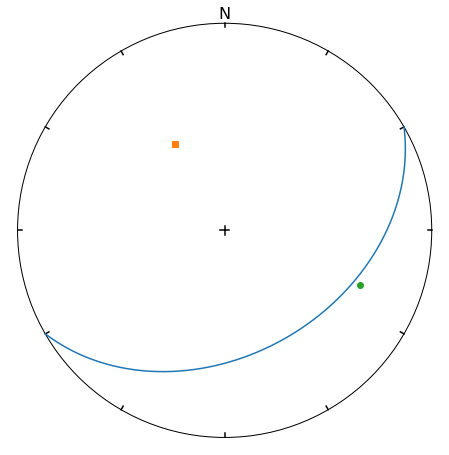When StereoNet class instance is created with arguments, they are immidiatelly plotted. Most of the objects provided by APSG could be plotted. Here we use the Group object and principal planes and lines of Ortensor:

In :
StereoNet(g, ot);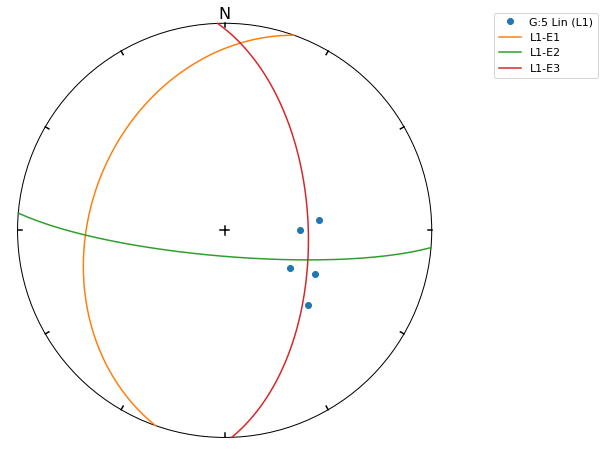A small circles (or cones) could be plotted as well

In :
s = StereoNet()
g = Group.randn_lin(mean=Lin(40, 15))
s.line(g, 'k.')
s.cone(g.R, g.fisher_stats['a95'], 'r')  # confidence cone on resultant
s.cone(g.R, g.fisher_stats['csd'], 'g')  # confidence cone on 63% of data
s.show()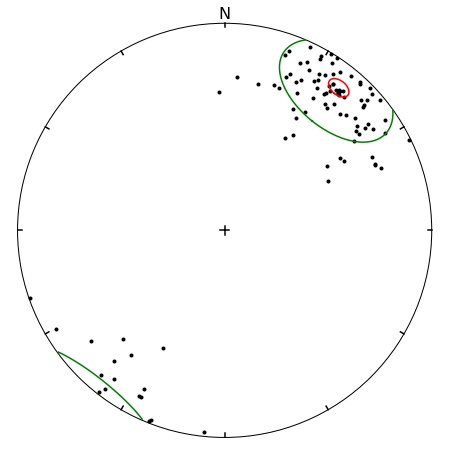To make density contours plots, a contour and contourf methods are available

In :
s = StereoNet()
g = Group.randn_lin(mean=Lin(40, 20))
s.contourf(g, 8, legend=True, sigma=2)
s.line(g, 'g.')
s.show()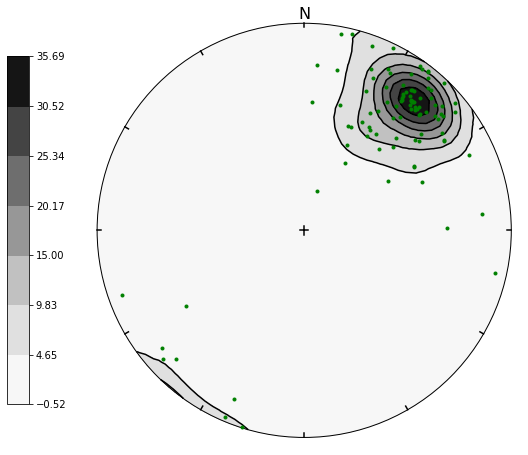Except Group, APSG provides PairSet and FaultSet classes to store Pair or Fault datasets. It can be inicialized by passing list of Pair or Fault objects as argument or use class methods from_array or from_csv

In :
p = PairSet([Pair(120, 30, 165, 20),
Pair(215, 60, 280,35),
Pair(324, 70, 35, 40)])
p.misfit

Out:
array([2.0650076 , 0.74600727, 0.83154705])
In :
StereoNet(p);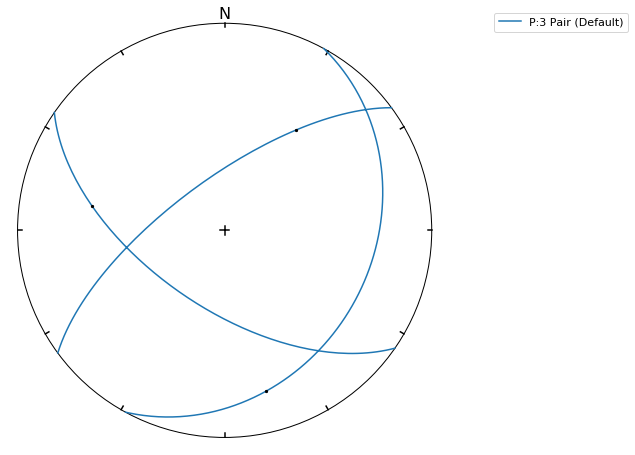StereoNet has two special methods to visualize fault data. Method fault produce classical Angelier plot

In :
f = FaultSet([Fault(170, 60, 182, 59, -1),
Fault(210, 55, 195, 53, -1),
Fault(10, 60, 15, 59, -1),
Fault(355, 48, 22, 45, -1)])
s = StereoNet()
s.fault(f)
s.line(f.p, label='P-axes')
s.line(f.t, label='T-axes')
s.plane(f.m, label='M-planes')
s.show()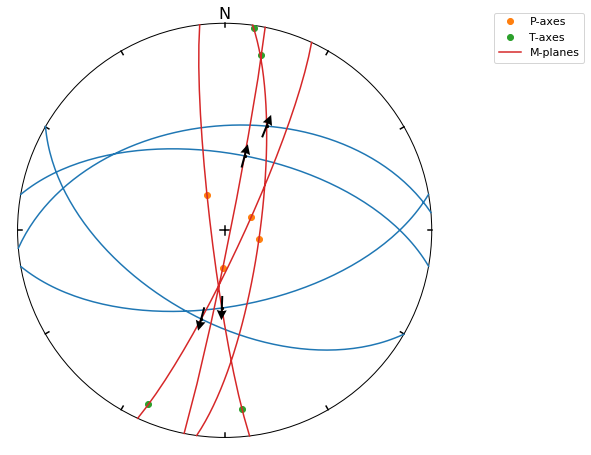hoeppner method produce Hoeppner diagram and must be invoked from StereoNet instance

In :
s = StereoNet()
s.hoeppner(f, label=repr(f))
s.show()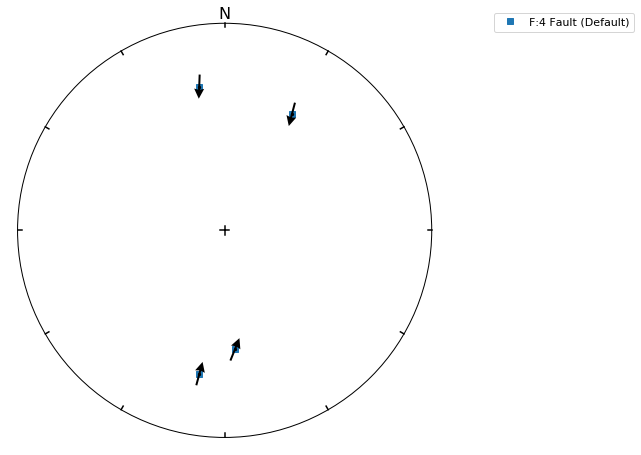Note that fault method is used, when data are passed directly to StereoNet instance

In :
f = Fault(120, 60, 110, 58, -1)
StereoNet(f, f.m, f.d, f.p, f.t);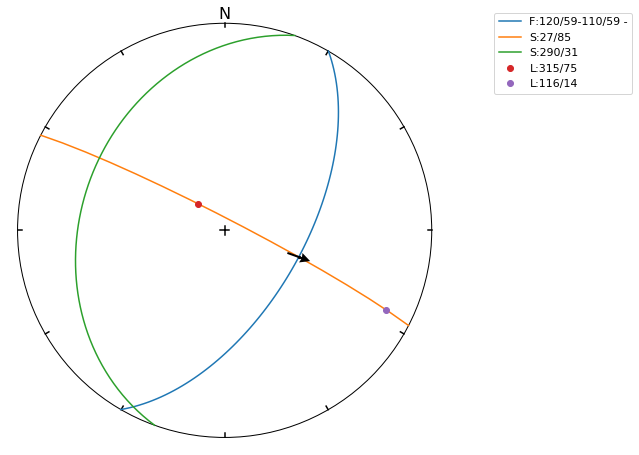## StereoGrid class¶

StereoGrid class allows to visualize any scalar field on StereoNet. Internally it is used for plotting contour diagrams, but it exposes apply_func method to calculate scalar field by any user-defined function. Function must accept three element numpy.array as first argument passed from grid points of StereoGrid.

Following example defines function to calculate resolved shear stress on plane from given stress tensor. StereoGrid is used to calculate this value over all directions and finally values are plotted by StereoNet

In :
S = Stress([[-10, 2, -3],[2, -5, 1], [-3, 1, 2]])
d = StereoGrid()
d.apply_func(S.shear_stress)
s = StereoNet()
s.contourf(d, 10, legend=True)
s.show()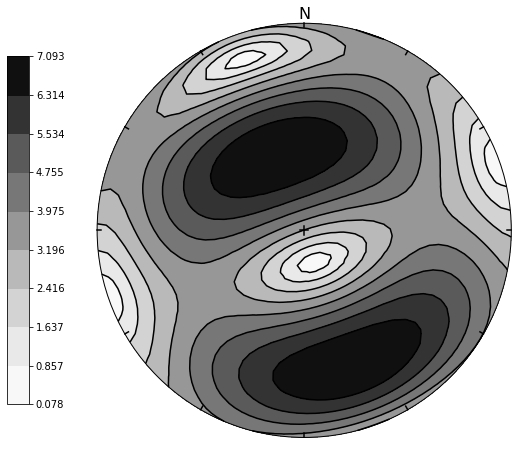The FaultSet provide also amgmech method which provide access to Angelier dihedra method. Results are stored in StereoGrid. Default behavior is to calculate counts (positive in extension, negative in compression)

In :
f = FaultSet.examples('MELE')
StereoNet(f.angmech());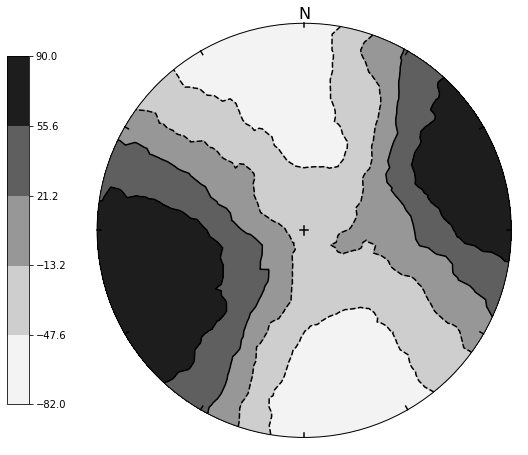Setting method to 'probability', maximum likelihood estimate is calculated.

In :
f = FaultSet.examples('MELE')
StereoNet(f.angmech(method='probability'));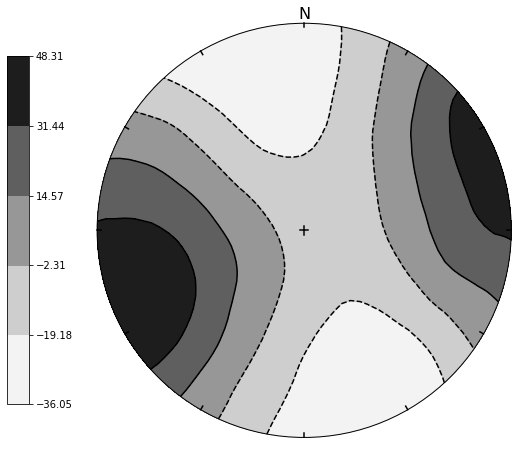## Fabric plots¶

Tensor-type objects (Ortensor, Ellipsoid) could be visualized in several specialized plots. FlinnPlot class provide classical Flinn's deformation diagram, RamsayPlot class provide Ramsay modification of Flinn's deformation diagram, VollmerPlot class provide triangular fabric plot (Vollmer, 1989) and HsuPlot class provide Hsu fabric diagram using natural strains.

In :
g1 = Group.examples('B2')
g2 = Group.examples('B4')
g3 = Group.uniform_lin(name='Uniform')

In :
FlinnPlot(g1, g2, g3);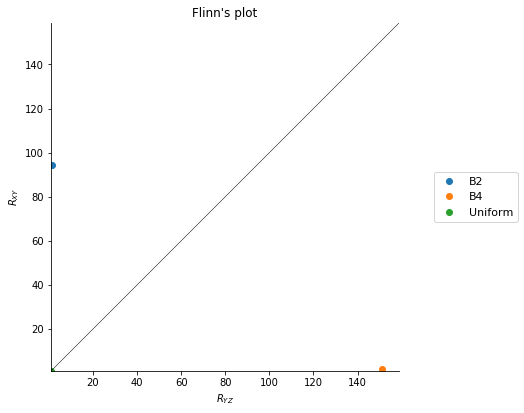In :
RamsayPlot(g1, g2, g3);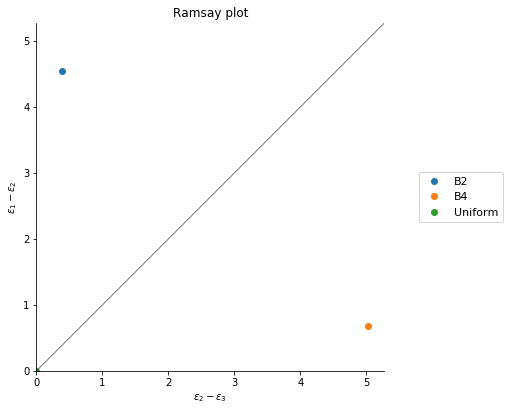In :
VollmerPlot(g1, g2, g3);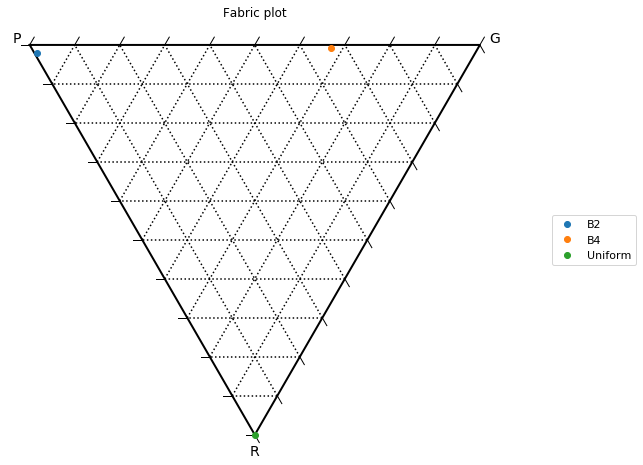In :
HsuPlot(g1, g2, g3);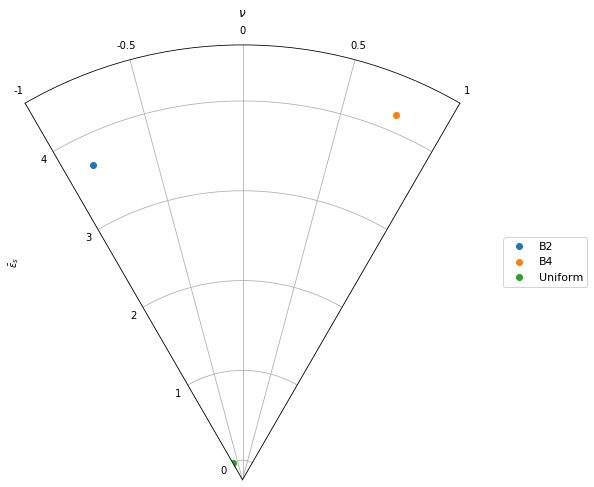All fabric plots has path method which accepts list of Tensor objects plotted as line.

In :
L = VelGrad.from_comp(xx=-2, zz=2)
Eevol = [E.transform(L.defgrad(t/50)) for t in range(50)]
r = FlinnPlot()
r.path(Eevol)
r.plot(E, 'ro')
r.show()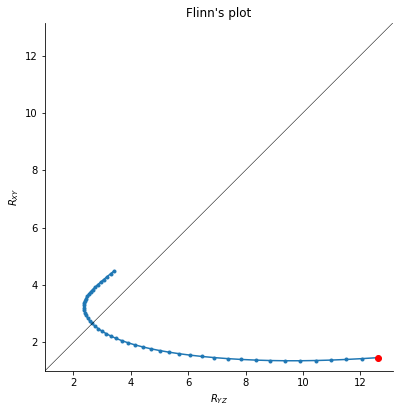In :
L = VelGrad.from_comp(xx=-2, zz=2)
Eevol = [E.transform(L.defgrad(t/50)) for t in range(50)]
r = RamsayPlot()
r.path(Eevol)
r.plot(E, 'ro')
r.show()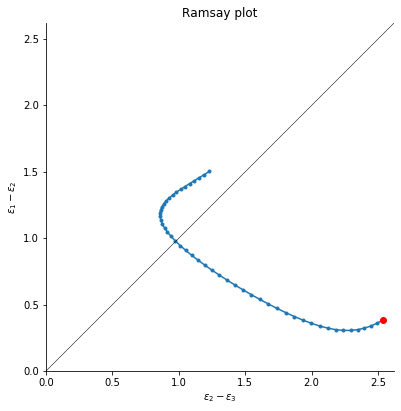In :
ot = g3.ortensor
otevol = [g3.transform(L.defgrad(t/50)).ortensor for t in range(50)]
f = VollmerPlot()
f.path(otevol)
f.plot(ot, 'ro')
f.show()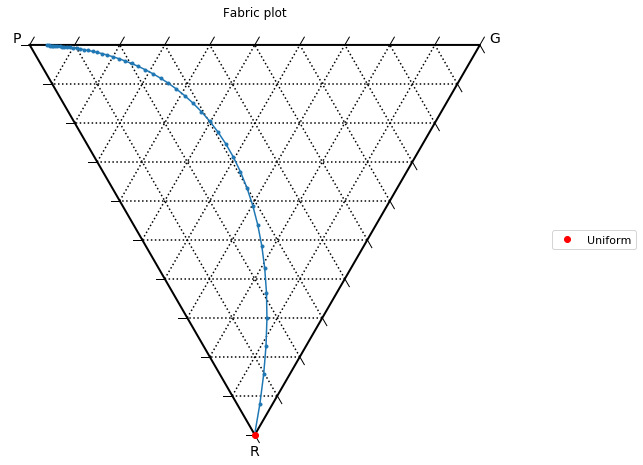In :
L = VelGrad.from_comp(xx=-2, zz=2)
Eevol = [E.transform(L.defgrad(t/50)) for t in range(50)]
r = HsuPlot()
r.path(Eevol)
r.plot(E, 'ro')
r.show()